# 2. The first step in glycolysis is catalyzed by the enzyme herokinase: glucose + ATP glucose-6-P...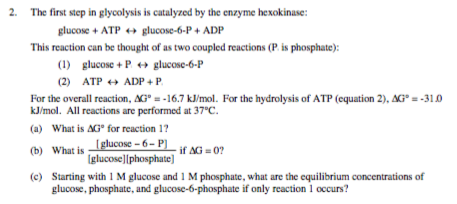2. The first step in glycolysis is catalyzed by the enzyme herokinase: glucose + ATP glucose-6-P + ADP This reaction can be thought of as two coupled reactions (P is phosphate): (1) glucose + P + glucose-6-P (2) ATP + ADP + P For the overall reaction, AG" = -16.7 kJ/mol. For the hydrolysis of ATP (equation 2), AG = -310 kJ/mol. All reactions are performed at 37°C. (a) What is AGº for reaction 1? Iglucose - 6-11 if AG 0? Iglucose)(phosphate) (c) Starting with 1M glucose and 1 M phosphate, what are the equilibrium concentrations of glucose, phosphate, and glucose-6-phosphate if only reaction l occurs? (b) What is glucose - 6-P)

∆G°overall = ∆G1° + ∆G2°

-16.7 kJ/mol = ∆G1° + (-31.0 kJ/mol)

∆G1° = (-16.7 + 31.0)kJ/mol

= +13.3 kJ/mol

If ∆G = 0,

Then ∆G° = -RTln(Keq)

Here, Keq = [glucose-6-phosphate]/[glucose][Pi]

T = 37°C = 37 + 273 = 310 K

R = 8.314 J/K mol

∆G° = +13.3 kJ/mol = +13300 J/mol

Substituting values,

+13300 J/mol = -(8.314 J/K mol)(310 K)ln(Keq)

ln(Keq) = +13300/(-2577.34) = -5.160

Keq = e-5.160 = 5.74 x 10-3

If only reaction 1 occurs, assuming reaction of 'x' mol each of glucose and phosphate, ICE table will be:

 [Glucose] [phosphate] [glucose-6-phosphate] Initial (M) 1 1 --- Change (M) -x -x +x Equilibrium (M) 1 - x 1 - x x

Keq = (x)/(1-x)(1-x) = 5.74 x 10-3

x/(1-x)2 = 0.00574

x = 0.00574 - 0.01148x - 0.00574x2

0.00574x2 + 1.01148x + 0.00574 = 0

On solving, x = 0.00567 M

So, the concentrations are:

[Glucose]= [phosphate] = 1-x

= 1- 0.00567 M = 0.994 M

[Glucose-6-phosphate] = x = 0.00567 M

#### Earn Coin

Coins can be redeemed for fabulous gifts.

Similar Homework Help Questions
• ### 1. 2. 3. 4. Select all that apply. Identify the reaction(s) of glycolysis inhibited by ATP □ glyceraldehyde-3-phosphate → 1,3-bisphosphoglycerate phosphoenolpyruvate +pyruvate 3-phosphoglycerate...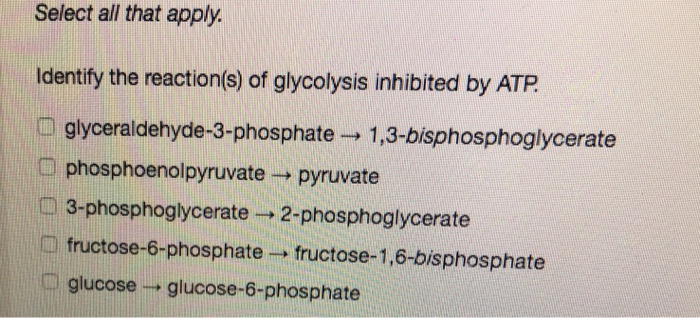1. 2. 3. 4. Select all that apply. Identify the reaction(s) of glycolysis inhibited by ATP □ glyceraldehyde-3-phosphate → 1,3-bisphosphoglycerate phosphoenolpyruvate +pyruvate 3-phosphoglycerate ~2-phosphoglycerate 1) fructose-6-phosphate→ fructose-1,6-bisphosphate . glucose glucose-6-phosphate Select all that apply. Alcohol fermentation: consumes ATP as it produces ethanol. O produces carbon dioxide begins as pyruvate is decarboxylated to acetaldehyde in a reaction catalyzed by alcohol dehydrogenase m regenerates NAD requires the enzyme alcohol dehydrogenase, In the figure below, what is the reaction occurring at the location...

• ### In the first step of glycolysis, the given two reactions are coupled. reaction 1: reaction 2:...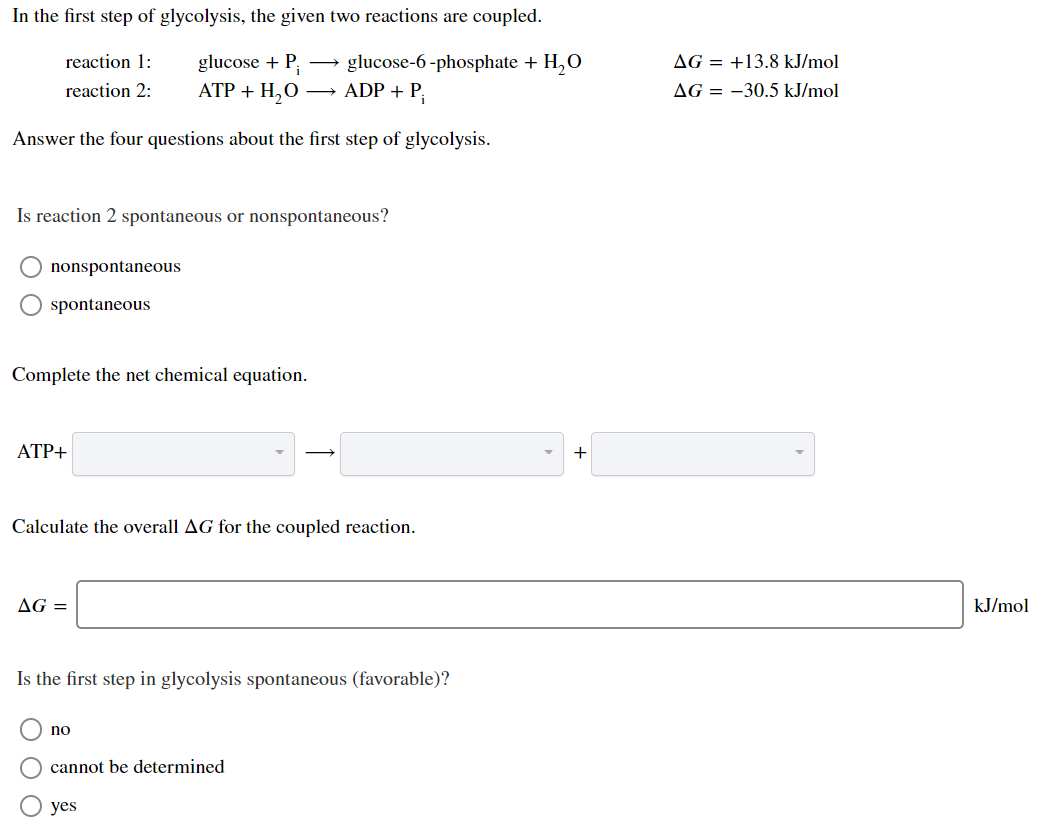In the first step of glycolysis, the given two reactions are coupled. reaction 1: reaction 2: glucose +P: ATP + H2O glucose-6-phosphate + H,O + ADP + P AG = +13.8 kJ/mol AG = -30.5 kJ/mol Answer the four questions about the first step of glycolysis. Is reaction 2 spontaneous or nonspontaneous? O nonspontaneous O spontaneous Complete the net chemical equation. ATP+ + Calculate the overall AG for the coupled reaction. AG = kJ/mol Is the first step in glycolysis...

• ### In the first step of glycolysis, the given two reactions are coupled... thank you! In the...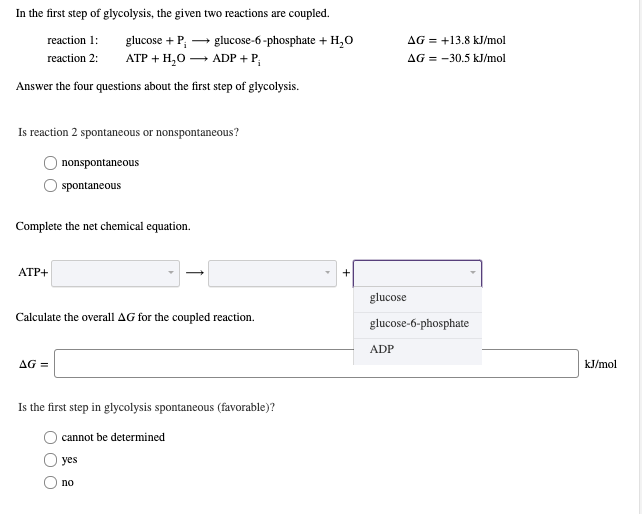In the first step of glycolysis, the given two reactions are coupled... thank you! In the first step of glycolysis, the given two reactions are coupled. reaction 1 glucose P АТР + H,О AG+13.8 kJ/mol AG 30.5 kJ/mol glucose-6-phosphate +H20 ADPP reaction 2: Answer the four questions about the first step of glycolysis Is reaction 2 spontaneous or nonspontaneous? nonspontaneous spontaneous Complete the net chemical equation АТР+ + glucose Calculate the overall AG for the coupled reaction. glucose-6-phosphate ADP AG...

• ### Hexokinase catalyzes the following reaction in glycolysis: Glucose + ATP ← → glucose-6-phosphate + ADP The...

Hexokinase catalyzes the following reaction in glycolysis: Glucose + ATP ← → glucose-6-phosphate + ADP The standard free-energy change for this reaction in the direction written is -16.7 kJ/mol. The concentrations of the related reaction components in the hepatocyte of a mammalian cell are: Glucose: 6 mM Glucose-6-phosphate: 0.074 mM ATP: 1.00 mM ADP: 0.25 mM What is the actual free-energy change for the reaction at body temperature (37 C)?

• ### Glycolysis is the process by which energy is harvested from glucose by living things. Several of...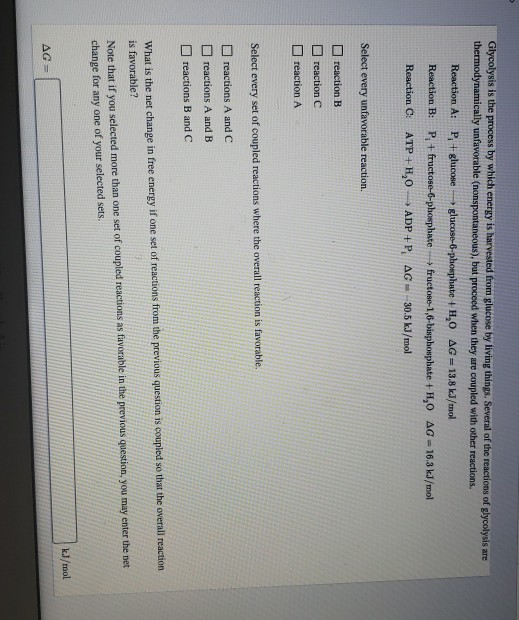Glycolysis is the process by which energy is harvested from glucose by living things. Several of the reactions of glycolysis are thermodynamically unfavorable (nonspontaneous), but proceed when they are coupled with other reactions. Reaction A: P + glucose glucose-6-phosphate +HO AG13.8 kJ/mol Reaction B: P fructose-6-phosphate + fructose-1,6-bisphosphate +1,0 AG 16.3 kJ/mol Reaction C: ATP+H, ADP +P, AG - 30.5 kJ/mol Select every unfavorable reaction. reaction B reaction C reaction A Select every set of coupled reactions where the overall...

• ### 1) SHOW WORK 12. During glycolysis, glucose 1-phosphate is converted to fructose 6-phosphate in two successive...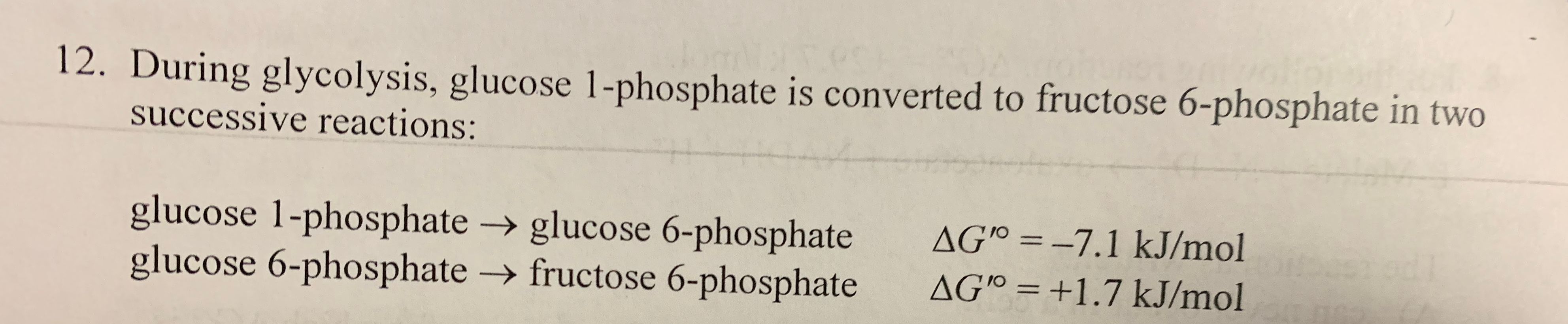1) SHOW WORK 12. During glycolysis, glucose 1-phosphate is converted to fructose 6-phosphate in two successive reactions: glucose 1-phosphate → glucose 6-phosphate glucose 6-phosphate + fructose 6-phosphate AG'' = -7.1 kJ/mol AG'º = +1.7 kJ/mol 13. The standard free-energy changes for the reactions below are given. phosphocreatine → creatine + Pi ATP → ADP + Pi AG" = 43.0 kJ/mol AG" = -30.5 kJ/mol What is the overall AG' for the following reaction? Iw a dee Hydrolysis of 1 M...

• ### The enzyme hexokinase catalyzes the following reaction: Glucose + ATP ↔ Glucose 6-phosphate + ADP For...

The enzyme hexokinase catalyzes the following reaction: Glucose + ATP ↔ Glucose 6-phosphate + ADP For this reaction, ΔG° = +16.7 kJ/mol a. Calculate the equilibrium constant for this reaction at 25 °C. Is this reaction spontaneous under standard conditions at this temperature? Calculate the ΔG if the reaction temperature was 25 °C and the concentrations are: glucose, 55.0 mM,; ATP, 5.00 mM; ADP, 1.00 mM; and glucose 6-Phosphate, 0.100 mM. Would the reaction be spontaneous under the conditions of...

• ### 16) In the reaction catalyzed by hexokinase, the two half reactions and their AGº values are...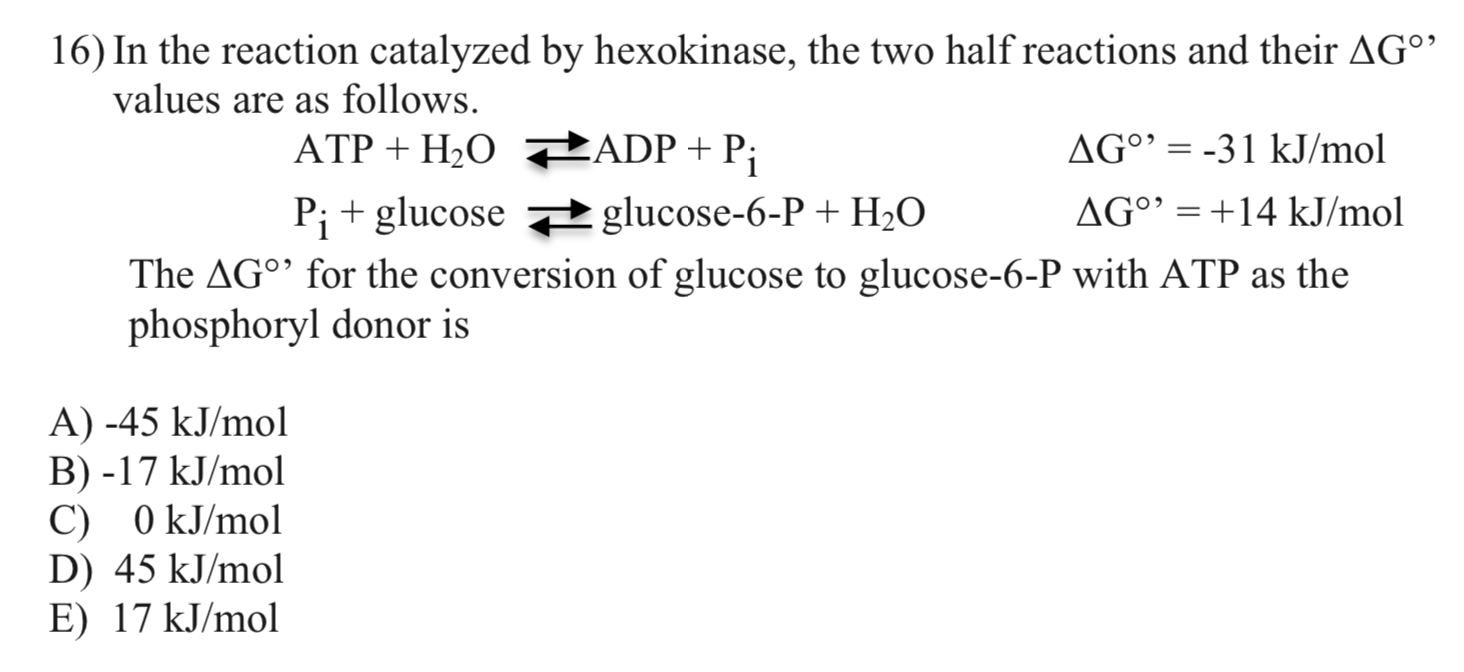16) In the reaction catalyzed by hexokinase, the two half reactions and their AGº values are as follows. ATP + H20 ADP + P; AG° = -31 kJ/mol Pi + glucose #glucose-6-P + H20 AG°' = +14 kJ/mol The AG°' for the conversion of glucose to glucose-6-P with ATP as the phosphoryl donor is A) -45 kJ/mol B) -17 kJ/mol C) 0 kJ/mol D) 45 kJ/mol E) 17 kJ/mol

• ### the enzyme hexokinase can catalyze the following reaction: fructose + ATP -> fructose 6 phosphate +...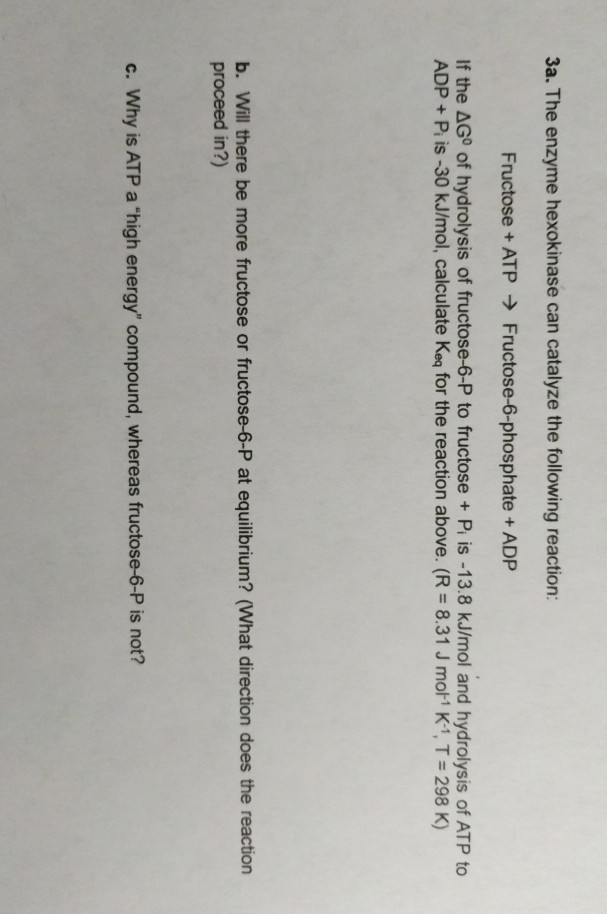the enzyme hexokinase can catalyze the following reaction: fructose + ATP -> fructose 6 phosphate + adp if the deltaG of hydrolysis of fructose 6 phosphate to fructose + Pi is -13.8 kj/mol and hydrolysis of atp to adp + pi is -30kj/mol, calculate Keq for the reaction above (R = 8.31 j mol-1 K-1, T = 298 k 3a. The enzyme hexokinase can catalyze the following reaction: Fructose + ATP → Fructose-6-phosphate + ADP If the ΔGo of hydrolysis...

• ### 1. Hexokinase catalyzes the ATP-dependent phosphorylation of a broad spectrum of 6- carbon sugars. In the...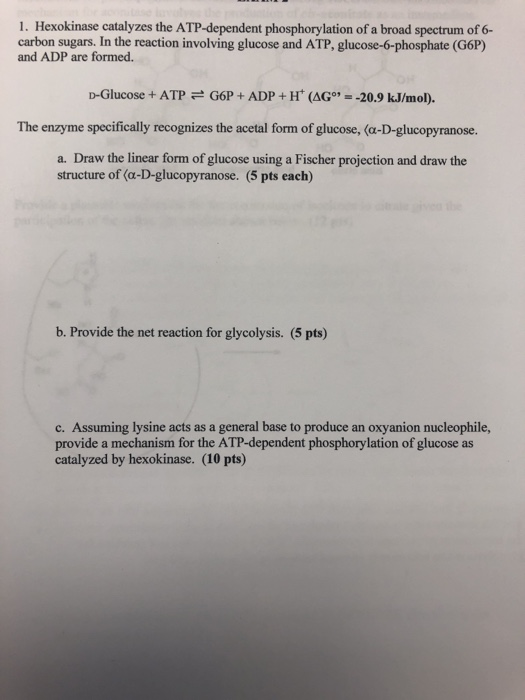1. Hexokinase catalyzes the ATP-dependent phosphorylation of a broad spectrum of 6- carbon sugars. In the reaction involving glucose and ATP, glucose-6-phosphate (G6P) and ADP are formed. D-Glucose + ATP G6P + ADP + Ht (AG",--20.9 kJ/mol). The enzyme specifically recognizes the acetal form of glucose, (a-D-glucopyranose. a. Draw the linear form of glucose using a Fischer projection and draw the structure of (a-D-glucopyranose. (5 pts each) b. Provide the net reaction for glycolysis. (5 pts) c. Assuming lysine acts...